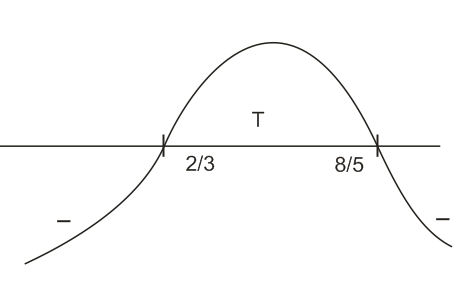Q

# Give answer! - Sequence and series - JEE Main-2

Let  be an A.P. with  . Then the

common difference of this A.P., which maximises the product

, is :

• Option 1)

• Option 2)• Option 3)

• Option 4)

Views

Assuming the first term of A.P. is a and difference is d.

Then,

Let

=>So,  will be maximum at

So, option (2) is correct.

Option 1)

Option 2)Option 3)

Option 4)

Exams
Articles
Questions